Examples from NCERT Book

Class 10
Chapter 12 Class 10 - Electricity

## (c) the total circuit resistance.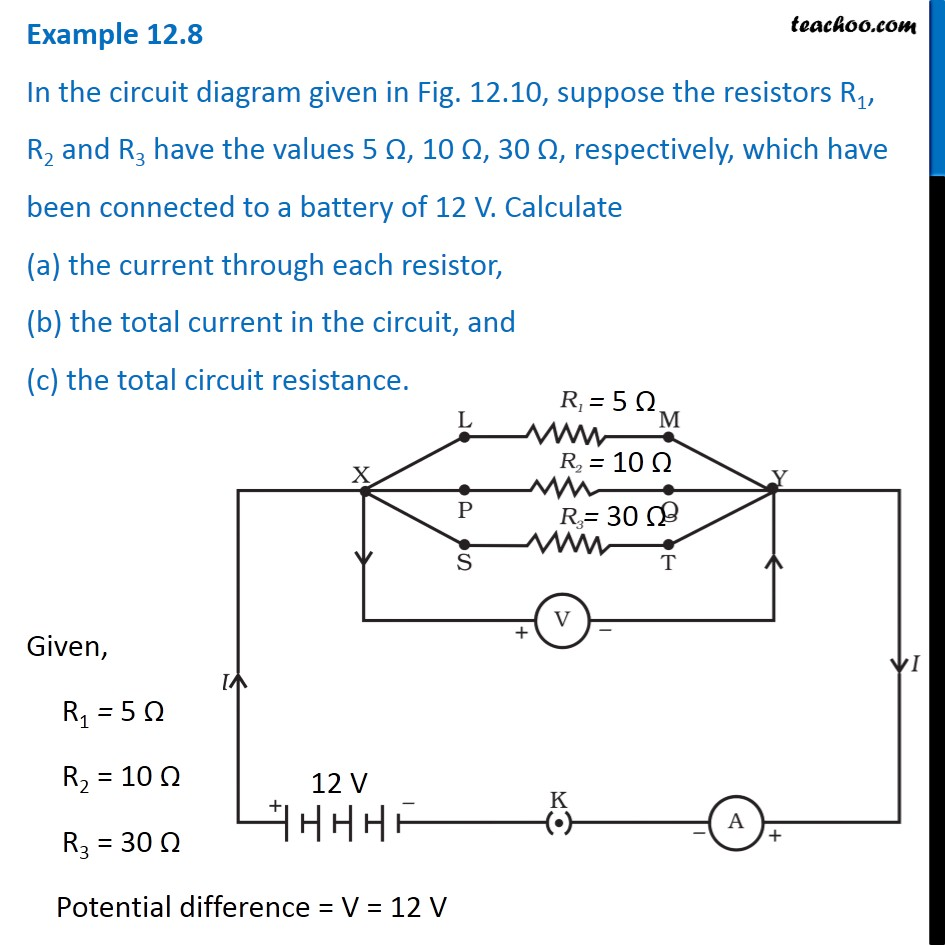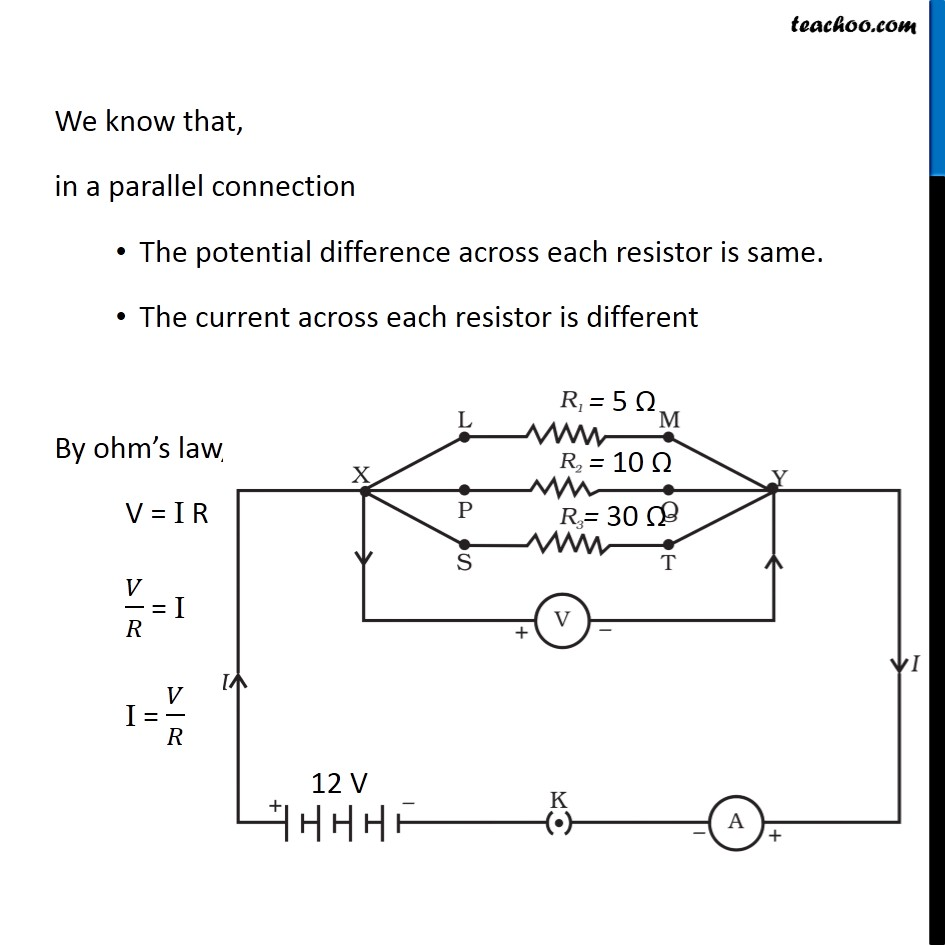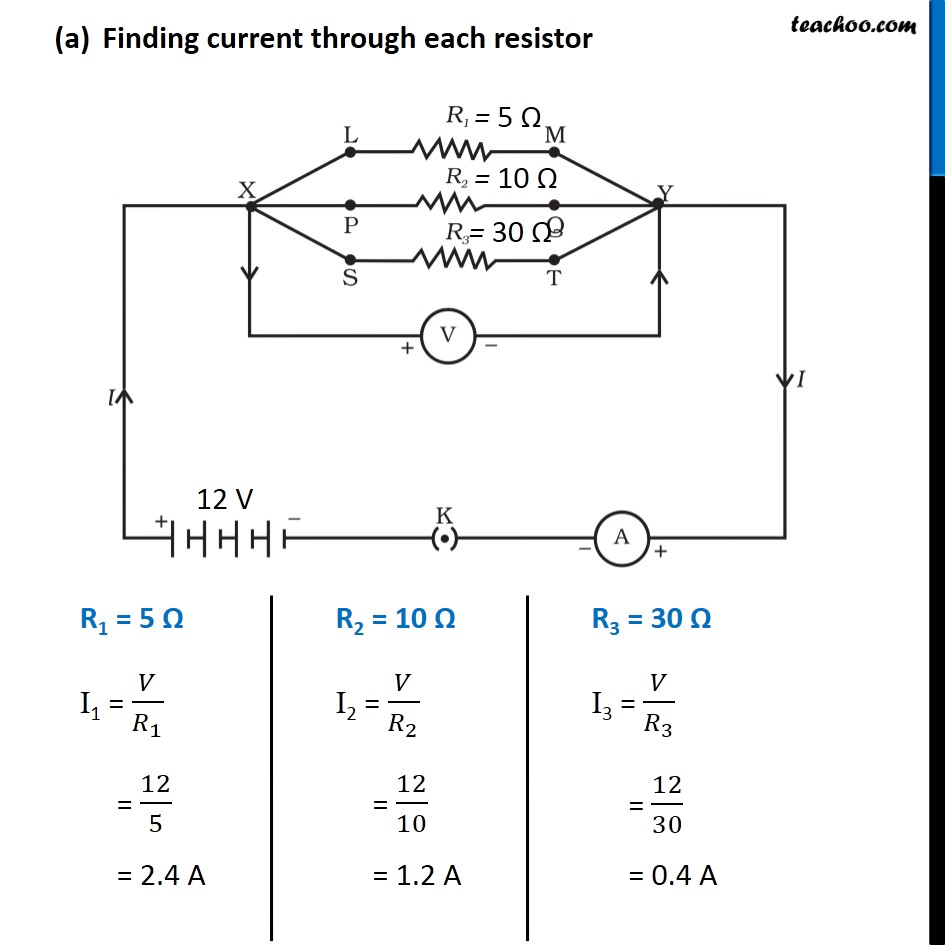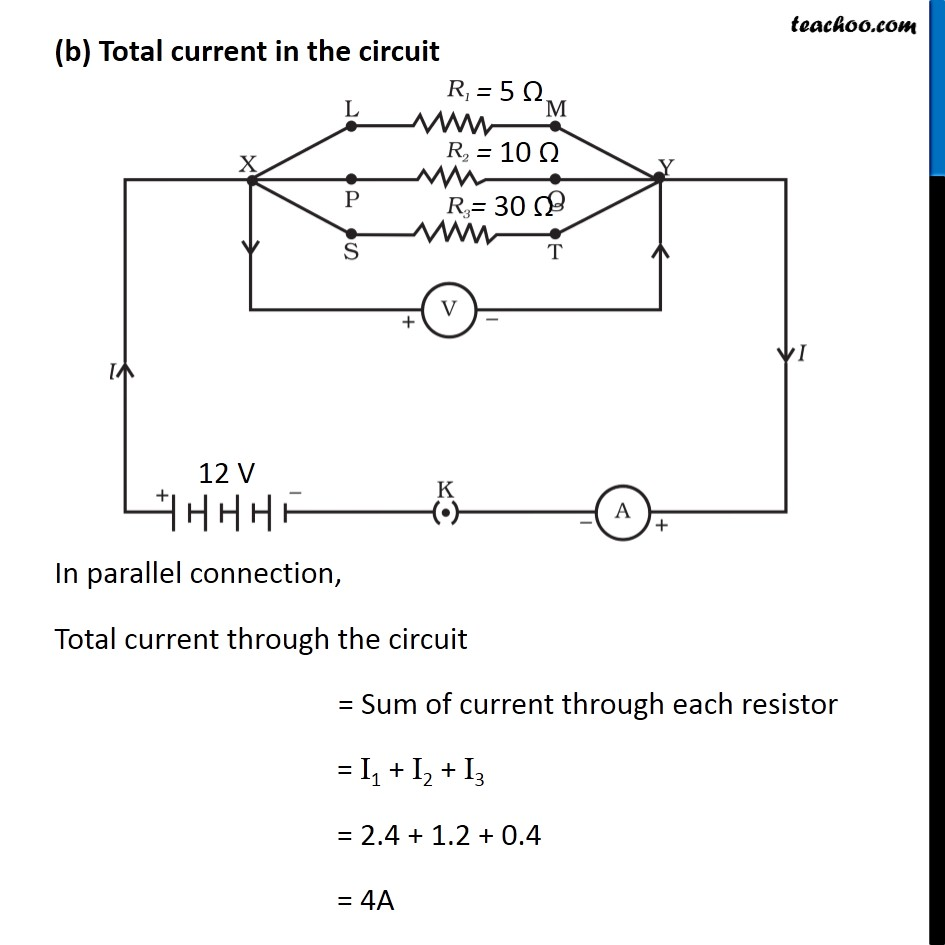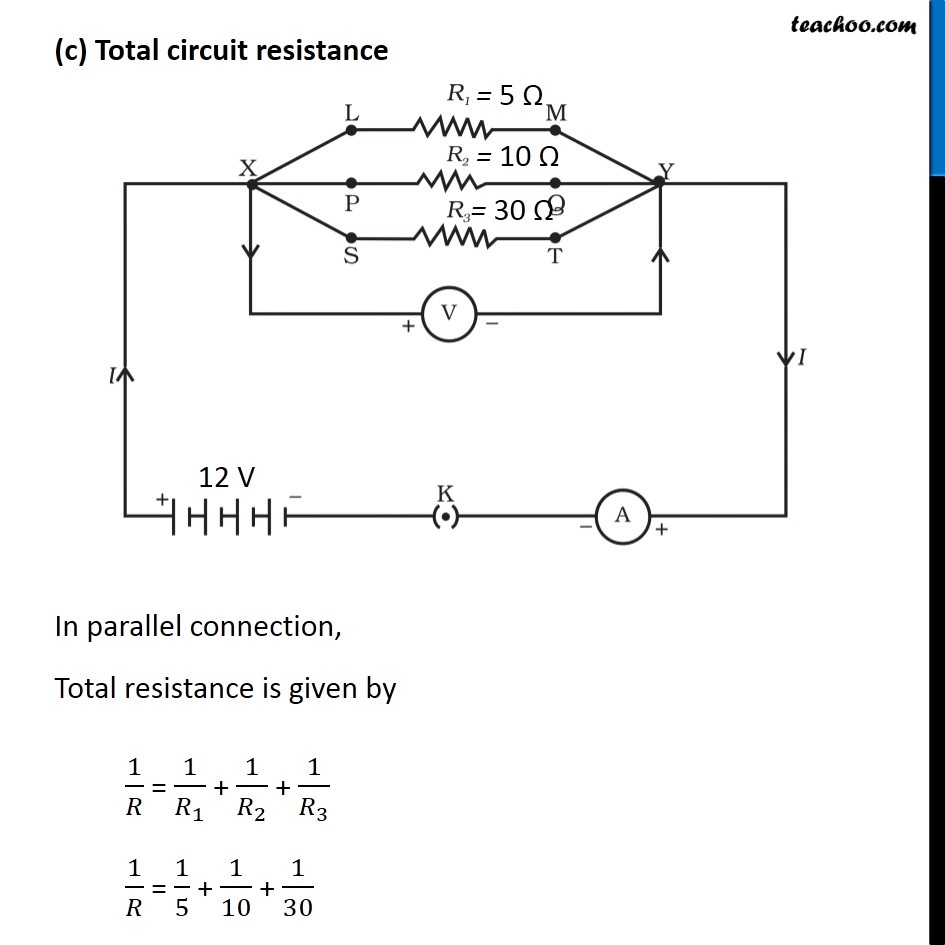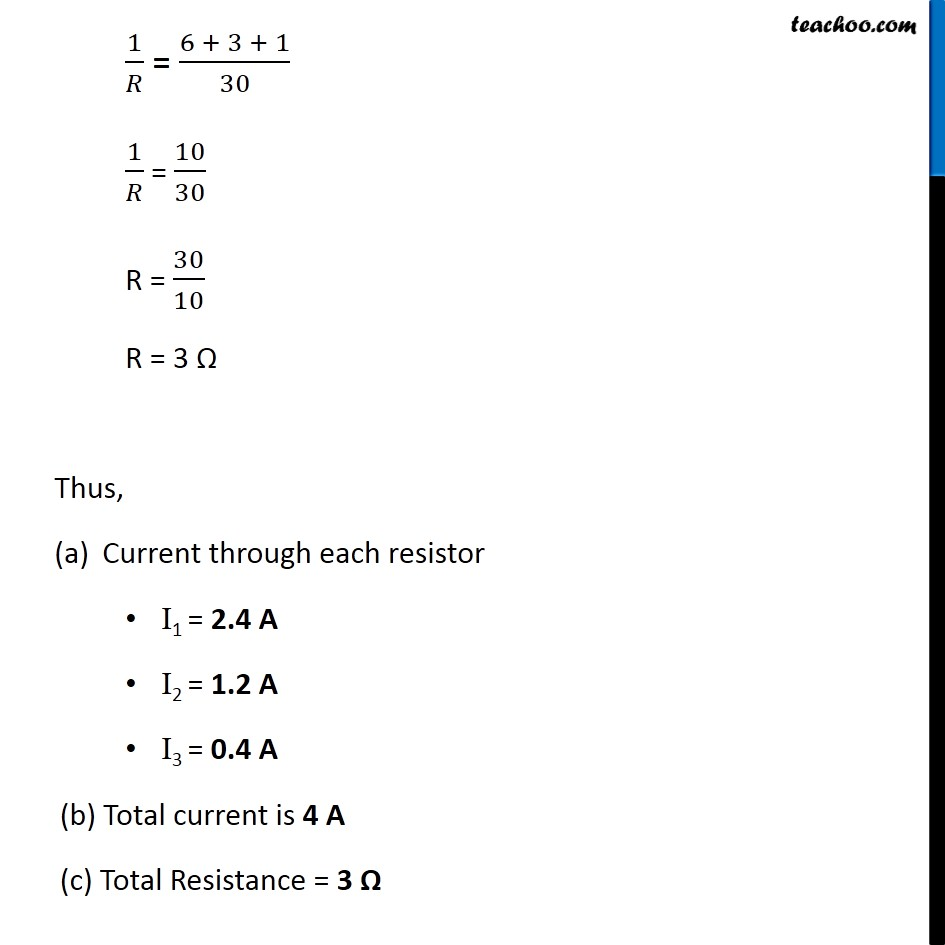Learn in your speed, with individual attention - Teachoo Maths 1-on-1 Class

### Transcript

Example 12.8 In the circuit diagram given in Fig. 12.10, suppose the resistors R1, R2 and R3 have the values 5 Ω, 10 Ω, 30 Ω, respectively, which have been connected to a battery of 12 V. Calculate (a) the current through each resistor, (b) the total current in the circuit, and (c) the total circuit resistance. Given, R1 = 5 Ω R2 = 10 Ω R3 = 30 Ω Potential difference = V = 12 V We know that, in a parallel connection The potential difference across each resistor is same. The current across each resistor is different By ohm’s law, V = I R 𝑉/𝑅 = I I = 𝑉/𝑅 Finding current through each resistor R1 = 5 Ω I1 = 𝑉/𝑅_1 = 12/5 = 2.4 A R2 = 10 Ω I2 = 𝑉/𝑅_2 = 12/10 = 1.2 A R3 = 30 Ω I3 = 𝑉/𝑅_3 = 12/30 = 0.4 A (b) Total current in the circuit In parallel connection, Total current through the circuit = Sum of current through each resistor = I1 + I2 + I3 = 2.4 + 1.2 + 0.4 = 4A (c) Total circuit resistance In parallel connection, Total resistance is given by 1/𝑅 = 1/𝑅_1 + 1/𝑅_2 + 1/𝑅_3 1/𝑅 = 1/5 + 1/10 + 1/30 1/𝑅 = (6 + 3 + 1)/30 1/𝑅 = 10/30 R = 30/10 R = 3 Ω Thus, Current through each resistor I1 = 2.4 A I2 = 1.2 A I3 = 0.4 A (b) Total current is 4 A (c) Total Resistance = 3 Ω